﻿ Measuring Time in microseconds? - Visual Studio - Netduino Forums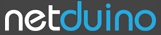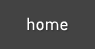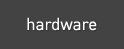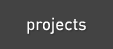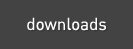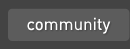The Netduino forums have been replaced by new forums at community.wildernesslabs.co. This site has been preserved for archival purposes only and the ability to make new accounts or posts has been turned off.# Measuring Time in microseconds?

2 replies to this topic

### #1Lain

Lain

Posted 03 March 2011 - 07:32 PM

Hi, I am new to netduino platform with Visual Studio 2010. I will like to know if there is a function for measuring time in microsecond. I am using the netduino to receive a square signal between 1 to 120 Hz through one of the analog pins. Then I make an interrupt with the rising/lower edge of the square signal. During the interruption I want to measure a starting time and then the stopping time so that I can measure the frequency of my signal. Note 1: Perhaps the problem can be solved with millisecond, but for more precise application, I would like to know if it is possible with this platform. This is because in other application I would like to do more processing than just getting the frequency of the signal (Ignition time for a motor for example). Note 2: I have tried using DateTime for trying to measure but it is not precise. Note 3: I haven’t found in visual studio the stopwatch function. That could be another possibility according to some forums.

### #2ItsDan

ItsDan

Posted 03 March 2011 - 09:28 PM

You can use the DateTime but you want to use the one passed to the interrupt function, because it's tagged with the time the interrupt occured and not the time the function got around to executing.

Total BOCS Traveled Distance: 9708 miles | States Visited: 5
Track the Box

### #3ItsDan

ItsDan

Posted 03 March 2011 - 09:33 PM

Actually I'm doing something similar. The following class was meant to measure similar signals. I plan to write up use cases for the project showcase but maybe it can be of use to you. I haven't optimized or thoroughly tested/simplified this yet.

Use:
```public static void Main()
{
while(true){}
}

static void reader_channelUpdated(ChannelInfo channel, float oldValue, float newValue)
{
Debug.Print(newValue.ToString());
}
```

Class:
```    /// <summary>
/// Represents the settings for a monitored channel
/// </summary>
class ChannelInfo
{
public InterruptPort pin;

//Retains the time in ticks the last time a change was
//observer on this channel
public long lastTick = -1;
public int channelID = -1;

//min- and max- pulse width values expected from the receiver
public int minValue = 1000;
public int maxValue = 2000;

//Stores the last reported value and minimum change required to
public float currentValue = -100.0f;
public float epsilon = 0.01f;
public int lastPulseWidth = -1;

//Negative range will return the value in the -1.0f - +1.0f range
//by default set to false for a 0.0f - +1.0f range
public bool negativeRange = false;

//If negative range is used this represents the neutral point, should
//normally be in the middle of the min/max range but could be tweaked
//during calibration
public int zeroPoint = -1;

//If clamp is set to true then values returned will NEVER exceed the
//expected bounds of the range
public bool clamp = true;
}

{
#region "Update Event"
public delegate void ChannelUpdated(ChannelInfo channel, float oldValue, float newValue);
public event ChannelUpdated channelUpdated;

protected virtual void OnChannelUpdate(ChannelInfo channel ,float oldValue, float newValue)
{
if (channelUpdated != null)
channelUpdated(channel, oldValue, newValue);
}
#endregion

//Constant to convert ticks to microseconds, appears to always be 10 on the Netduino
private const long ticksToMicroseconds = (long)(TimeSpan.TicksPerMillisecond / 1000);
public ChannelInfo[] channels;
public bool isCalibrating = false;

{
//Initialize the channel array, uses a mostly-empty array
//to avoid costly lookups of elements with the trade-off
channels = new ChannelInfo;
}

/// <summary>
/// Begins monitoring a channel on the receiver given a pin index
/// </summary>
/// <param name="pinIndex">Index of the digital pin to monitor for this channel</param>
/// <param name="channelID">ID number of the receiver channel this pin represents</param>
public ChannelInfo addChannel(Cpu.Pin pin, int channelID)
{
ChannelInfo c = new ChannelInfo();
c.channelID = channelID;
c.pin = new InterruptPort(pin, false, Port.ResistorMode.Disabled, Port.InterruptMode.InterruptEdgeBoth);
c.pin.OnInterrupt += new NativeEventHandler(port_OnInterrupt);

channels[(int)pin] = c;
return c;
}

/// <summary>
/// Retrieves the ChannelInfo object for a given pin
/// </summary>
/// <param name="pin"></param>
/// <returns></returns>
public ChannelInfo getChannel(Cpu.Pin pin)
{
return channels[(int)pin];
}

/// <summary>
/// Called when an intterupt event occurs on one of the monitored pins
/// </summary>
private void port_OnInterrupt(uint data1, uint state, DateTime time)
{
ChannelInfo channel = channels[(int)data1];

if (state == 0)
{
long pulseWidth = time.Ticks - channel.lastTick;
int pulseWidthMicroseconds = (int)(pulseWidth / ticksToMicroseconds);
pulseMeasured(channel, pulseWidthMicroseconds);
}
else
{
channel.lastTick = time.Ticks;
}
}

private void pulseMeasured(ChannelInfo channel, int microseconds)
{
float val = convertPulseToRange(channel, microseconds);

if (isCalibrating)
{
channel.maxValue = System.Math.Max(channel.maxValue, microseconds);
channel.minValue = System.Math.Min(channel.minValue, microseconds);
}
else
{
if (floatAbs(val - channel.currentValue) > channel.epsilon)
{
float cVal = channel.currentValue;
channel.currentValue = val;
OnChannelUpdate(channel, cVal, val);
}

channel.lastPulseWidth = microseconds;
}
}

/// <summary>
/// Converts a measured pulse-width to a range based on the channel's settings
/// </summary>
private float convertPulseToRange(ChannelInfo channel, int microseconds)
{
float val = 0.0f;

if (channel.zeroPoint == -1)
{
val = (float)(microseconds - channel.minValue) / (float)(channel.maxValue - channel.minValue);

if (channel.negativeRange)
val = (val - 0.5f) * 2.0f;
}
else
{
if (microseconds > channel.zeroPoint)
{
val = (float)(microseconds - channel.zeroPoint) / (float)(channel.maxValue - channel.zeroPoint);
}
else
{
val = -1 * ((float)(channel.zeroPoint - microseconds) / (float)(channel.zeroPoint - channel.minValue));
}
}

if (channel.clamp)
val = clamp(val, channel.negativeRange);

return val;
}

#region "Calibration"

/// <summary>
/// Begin calibration mode. Min/Max values are recorded, zeroPoint is set to current state,
/// and no update events will fire. All RC controls should be in neutral position.
/// </summary>
public void startCalibration()
{
isCalibrating = true;

ChannelInfo c;
for (int i = 0; i < channels.Length; i++)
{
c = channels[i];
if (!(c == null))
{
c.zeroPoint = c.lastPulseWidth;
c.maxValue = c.lastPulseWidth+1;
c.minValue = c.lastPulseWidth-1;
}
}
}

/// <summary>
/// End calibration mode and resume event firing
/// </summary>
public void endCalibration()
{
isCalibrating = false;

ChannelInfo c;
for (int i = 0; i < channels.Length; i++)
{
c = channels[i];
if (!(c == null))
{
Debug.Print("obj.channels[" + c.pin.Id.ToString() + "].minValue = " + c.minValue.ToString() + ";");
Debug.Print("obj.channels[" + c.pin.Id.ToString() + "].maxValue = " + c.maxValue.ToString() + ";");
Debug.Print("obj.channels[" + c.pin.Id.ToString() + "].zeroPoint = " + c.zeroPoint.ToString() + ";");
Debug.Print("");
}
}
}
#endregion

/// <summary>
/// Clamps the supplied value to the specified range
/// </summary>
private float clamp(float val, bool negativeRange)
{
if (val > 1.0f) val = 1.0f;

if (negativeRange)
{
if (val < -1.0f) val = -1.0f;
}
else
{
if (val < 0.0f) val = 0.0f;
}

return val;
}

/// <summary>
/// Apparently there's no Abs() overload in the System.Math class
/// for float values.
/// </summary>
/// <param name="x"></param>
/// <returns></returns>
private float floatAbs(float x)
{
if (x < 0) return -1.0f * x;
return x;
}
}
```

Total BOCS Traveled Distance: 9708 miles | States Visited: 5
Track the Box

#### 0 user(s) are reading this topic

0 members, 0 guests, 0 anonymous usershome    hardware    projects    downloads    community    where to buy    contact Copyright © 2016 Wilderness Labs Inc.  |  Legal   |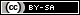This webpage is licensed under a Creative Commons Attribution-ShareAlike License.